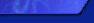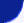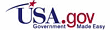Home About Us Contact Us SearchData Access

Classic Maps

Program Info

Publications# What averaging procedures are performed on the wind measurements?

Two wind averaging methods are used to calculate average wind speed and direction.

1. The first wind averaging technique applies to those measurements reported by all DACT, VEEP, and ARES payloads. The average wind speed is the simple scalar average of the wind speed observations. A "unit-vector" average is used to calculate the average wind direction. In this technique, unity serves as the length of the vector, and the wind direction observations serve as the orientation of the vector. The u and v components are then calculated for each observation. Next, the average u and v components are computed and the average wind direction is derived from "arctan(u/v)." Note that this technique will produce greater wind speeds than if a true vector average was used.
2. The second method, used to calculate average speed and direction reported by NDBC's older GSBP payloads, is a true vector average. In this scheme, the magnitude of the vector is represented by the wind speed observation and the direction observations are used for the orientation. The vectors are then broken down into their u and v components. All u and v components are then averaged separately. The resulting average speed and direction are calculated from the Pythagorean Theorem and "arctan(u/v)," respectively.
For a comparison of vector and scalar averaging of winds, see:

Gilhousen, D.B., 1987: A field evaluation of NDBC moored buoy winds. Journal of Atmospheric and Oceanic Technology,4, 94-104.